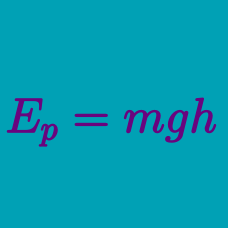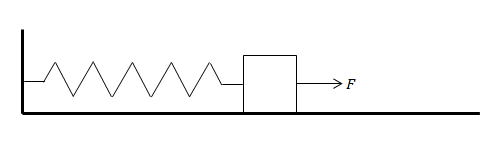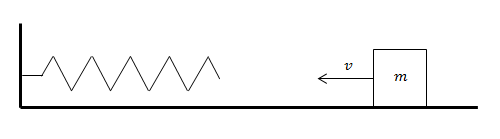Classical Mechanics

# Potential energy of springs

A spring is shortened by $2\text{ m}$ from its original length. If the elastic potential energy of the spring is $10\text{ J},$ what is the value of the spring constant?

A spring hanging from the ceiling has a length of $4\text{ m},$ and its spring constant is $k=20\text{ N/m}.$ If the spring is pulled so that its length gets doubled, what is the elastic potential energy in the spring?An object is at rest on a frictionless surface is attached to the wall by an elastic spring of strength $\SI[per-mode=symbol]{10}{\newton\per\meter}.$

A force of strength $F=40\text{ N}$ pulls the object toward the right.

What is the elastic potential energy of the spring at equilibrium?In the figure above, an object of mass $m=4\text{ kg}$ is sliding towards a spring with a speed of $v=6\text{ m/s}$ on a frictionless horizontal floor. If the spring constant is $k=1\text{ N/m},$ what is the maximal change in the spring's length?

The spring in a monster truck's suspension is shortened by $4\text{ m}$ from its original length. If the spring constant is $k=2\text{ N/m},$ what is the elastic potential energy of the spring?

×